# Inverting the Discrete Cosine Transform¶

To demonstrate the use of Grackle in the analysis of real world programs, we have applied it (and our custom ILP solver BLT) to the problem of computing preimages from JPEG decompression. Specifically, given constraints on pixel values in a target image and specific JPEG quality level, the problem is to find an input that will create this image.

This problem can be naturally encoded as a linear constraint problem over integers. When compressing an image, JPEG performs the following steps:

• The image is transformed from its current color space (such as RGB) into a color space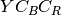which consists of a luminance (brightness) channel and two chrominance channels. For monochrome images, only the luminance channel is needed. Each channel uses a 8-bit integer to encode the value at each pixel, which results in pixel values ranging from 0 to 255. However, JPEG internally subtracts 128 from each coefficient value in later steps so that they are centered on zero. For the luminance channel, this menas that -128 represents black, and 127 represents white.

• The two chrominance channels are optionally down-sampled, so that each 2x2 block of pixel values is collapsed to a single pixel or a 2x1 row of pixels. The human eye is less sensitive to find-grained changes to hue and saturation than changes to overall brightness, and so those channels can be downsampled with less of an impact to image quality.

• Within each of the color channels, the image is split into 8x8 blocks. If a channel contains a number of rows or columns that is not a multiple of 8, then padding is added to the image. The extra pixels values are unspecified and will be discarded during decompression, and a typical choice may be to use the average value of the existing pixels.

• A 2-d discrete cosine transform is applied to each 8x8 block that transforms the coordinate space from the image pixel values to the frequency domain. This has the effect of separating out the image components by frequency, so that course-grained qualities such as overall brightness are represented distinctly from more fine-grained fluctuations such as texture. For a given input block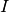, we denote the frequency representation by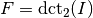.

• A quantization step is performed. This rounds each coordinate in the frequency domain to the nearest multiple of an associated value in specific JPEG quantization matrix, which is a function of the JPEG quality level, an integer ranging from 1 to 100, and also depends on whether this is a luminance or chrominance channel. We denote the luminance quantization matrix at quality levelby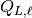, and corresponding chrominance quantization matrix by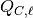.

When perceiving images, the human eye is much less perceptive of high-frequency changes than low-frequency changes in the image. The quantization matrix is been tailored to take advantage of this and minimize the perceived loss of information. For example, we show the quantization matrix at level 50 below:

ans =

16    11    10    16    24    40    51    61
12    12    14    19    26    58    60    55
14    13    16    24    40    57    69    56
14    17    22    29    51    87    80    62
18    22    37    56    68   109   103    77
24    35    55    64    81   104   113    92
49    64    78    87   103   121   120   101
72    92    95    98   112   100   103    99


In the table, the bottom right corresponds to high-frequency components while the top-left corresponds to low-frequency components. The fact that quantization is more course at fine grained components is reflected in the matrix by the fact that the coefficients in the higher-frequency components are much larger than the coefficients in the low-frequency components. At this quality level, many of the cofficients at more fine-grained coefficients will be 0 due to rounding.

Given the output of the frequency transform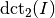, the output of the quantization step consists of the rounded quotient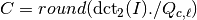. The division is a pointwise (Hadamard) division, rather than an inverse linear transform.

• Finally, a variant of Huffman compression is performed that compresses the quantized coefficients into a string of bits. The compression is lossless, and designed to represent the quantized coefficients in a small number of bits.

For the preimage problem, we are interested in decompression rather than compression, and so the above steps are performed in reverse order. For the sake of illustration, we focus here on preimages of greyscale images, so this allows us to ignore steps (1) and (2). We can also ignore the Huffman compression step, as it is lossless and easily inverted. Note that we have also investigated and solved the color image version of this problem. The resulting encodings are larger than those for greyscale images, but structurally similar.

Overall then, decompression can be modeled as inverting quantization followed by an inverse DCT: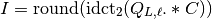The extra rounding is needed to convert the real values from the inverse DCT operation to integer values for the image.

As an example, suppose that we are looking for an image containing Hello World! at specific pixel locations. Within Matlab, we encode the constraints as an cell array of strings, which contains either the value we care about, or 'xx' in locations where the output does not matter.

P = { 'xx' 'xx' 'xx' 'xx' 'xx' 'xx' 'xx' 'xx'
'xx' 'xx' 'xx' 'xx' 'xx' 'xx' 'xx' 'xx'
'xx' 'xx' 'xx' 'xx' 'xx' 'xx' 'xx' 'xx'
'xx' 'xx' 'xx' 'xx' 'xx' 'xx' 'xx' 'xx'
'xx' 'xx' 'xx' 'xx' 'xx' 'xx' 'xx' 'xx'
'xx' '48' '65' '6c' '6c' '6f' '20' 'xx' % "Hello "
'xx' '57' '6f' '72' '6c' '64' '21' 'xx' % "World!"
'xx' 'xx' 'xx' 'xx' 'xx' 'xx' 'xx' 'xx' };


To model this question as a bounded ILP problem, we make two approximations that have had minimal impact in practice. We first approximate the real values returned by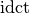with rational fractional values with 28 bits of precision.

We then compute lower and upper bounds from the matrix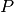. For entries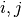where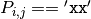, we let the lower and upper bounds be the minimum and maximum value for pixels (i.e., 0 and 255.5 respectively). For the other entries where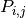is defined, we currently obtain bounds by subtracting 128 from each coordinate to accommodate the shift and then subtracting and adding 0.5 to obtain lower and upper bounds respectively. For the matrixabove, this results in the following lower (L) and upper (H) bounds:

L =

-128.5 -128.5 -128.5 -128.5 -128.5 -128.5 -128.5 -128.5
-128.5 -128.5 -128.5 -128.5 -128.5 -128.5 -128.5 -128.5
-128.5 -128.5 -128.5 -128.5 -128.5 -128.5 -128.5 -128.5
-128.5 -128.5 -128.5 -128.5 -128.5 -128.5 -128.5 -128.5
-128.5 -128.5 -128.5 -128.5 -128.5 -128.5 -128.5 -128.5
-128.5  -56.5  -27.5  -20.5  -20.5  -17.5  -96.5 -128.5
-128.5  -41.5  -17.5  -14.5  -20.5  -28.5  -95.5 -128.5
-128.5 -128.5 -128.5 -128.5 -128.5 -128.5 -128.5 -128.5

H =

127.5  127.5  127.5  127.5  127.5  127.5  127.5  127.5
127.5  127.5  127.5  127.5  127.5  127.5  127.5  127.5
127.5  127.5  127.5  127.5  127.5  127.5  127.5  127.5
127.5  127.5  127.5  127.5  127.5  127.5  127.5  127.5
127.5  127.5  127.5  127.5  127.5  127.5  127.5  127.5
127.5  -55.5  -26.5  -19.5  -19.5  -16.5  -95.5  127.5
127.5  -40.5  -16.5  -13.5  -19.5  -27.5  -94.5  127.5
127.5  127.5  127.5  127.5  127.5  127.5  127.5  127.5


The problem is now reduced to finding coefficients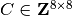such that: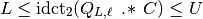As both the Hadamard product and inverse-DCT are linear transformations, this can be easily converted into an ILP problem.

Now that we have a bounded ILP problem, we can apply SMT solving or BLT to these problems.

Here we describe a top-level scipt for solving the problem using Grackle.

% Set the default solver to BLT (capable of handling bounded ILP)
mss_config('default_solver', 'blt');

% Use 28 bits of precision
mss_config('blt_params', ['-s ' num2str(2^28)]);

% Generate 8x8 symbolic integer coefficients
coef = symbolic('coef', 8, 8, 'integer')

% Set the quantization levels the we attempt to solve under. Note: the
% level has a significant effect on the satisfiability of the problem.
% Levels 27 and above are known to be SAT whereas levels 18 and
% below are known to be UNSAT.
lvl = 50;

constraints = { 'xx' 'xx' 'xx' 'xx' 'xx' 'xx' 'xx' 'xx'
'xx' 'xx' 'xx' 'xx' 'xx' 'xx' 'xx' 'xx'
'xx' 'xx' 'xx' 'xx' 'xx' 'xx' 'xx' 'xx'
'xx' 'xx' 'xx' 'xx' 'xx' 'xx' 'xx' 'xx'
'xx' 'xx' 'xx' 'xx' 'xx' 'xx' 'xx' 'xx'
'xx' '48' '65' '6c' '6c' '6f' '20' 'xx' % Hello
'xx' '57' '6f' '72' '6c' '64' '21' 'xx' % World
'xx' 'xx' 'xx' 'xx' 'xx' 'xx' 'xx' 'xx' };

[l,h] = bounds(0, 255, constraints);

q = luminance_quantization_matrix(lvl);
img = my_idct2(q .* coef);

% assert that the image satisfies our constraints
p = l <= img & img <= h;

% call the external solver to find a satisfying assignment
[r,c] = check_sat(p);

if r == 1
msg = 'Checking solution...'
c.coef;
y = my_idct2(q .* c.coef);
o = l <= y & y <= h;
test_check(o);
else
msg = 'No solution found...'
end


The functions bounds and luminance_quantization_matrix are as described above. Our implementation of the 2-d IDCT is the following:

function r = my_idct2(x)
N  = length(x);

for c = 1:N
x(:,c) = idct(x(:,c));
end

for r = 1:N
x(r,:) = idct(x(r,:));
end

r = x;
end


Similarly, the 1-d IDCT is:

function r = idct(x)
N = length(x);

w =sqrt(2/N);
for k = 1:N

c(1) = sqrt(1/N);
for i = 2:N
c(i) = w*cos(pi * (i-1) * (2*k-1)/(2*N));
end
r(k) = dot(c,x);
end
end


It’s worth noting that the coefficients of the form w*cos(..) are captured in exact form by the symbolic simulator. When the problem is written out to an external solver that does not handle exact real valued constants (e.g. Yices, or BLT) the coefficients are approximated using 64-bit floating point values.